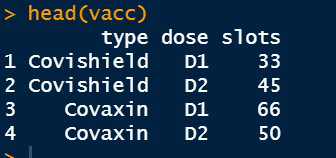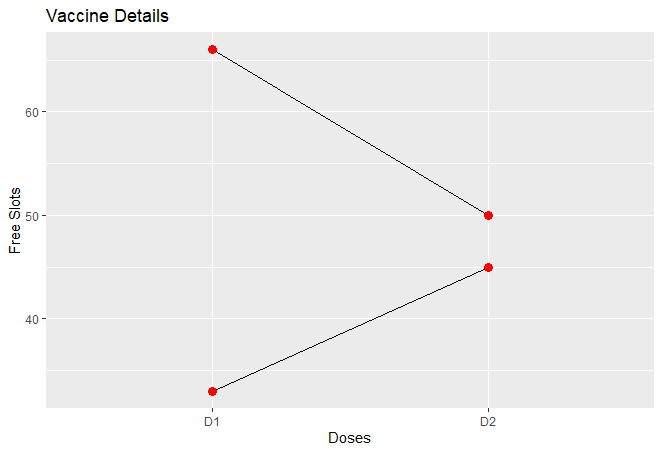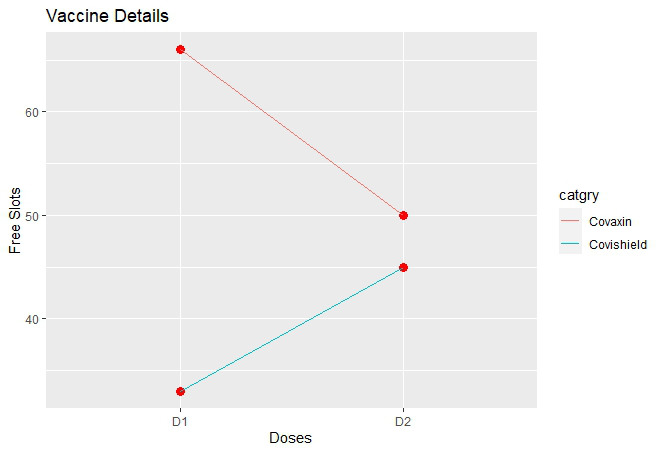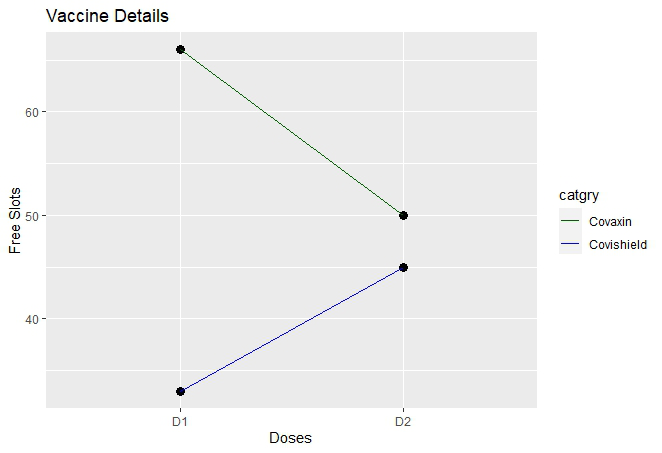Related Articles

# Add legend for multiple lines in R using ggplot2

• Last Updated : 24 Jun, 2021

In this article, we are going to see how to add legends for multiple line plots in R Programming Language using ggplot2. First, you need to install the ggplot2 package if it is not previously installed in R Studio.

The functions used to create the line plots are :

• geom_line( ) : To plot the line and assign its size, shape, color, etc.

Syntax:

geom_line(mapping=NULL, data=NULL, stat=”identity”, position=”identity”,…)

• geom_point( ) : It is used to add points to the end of the lines. It is used to assign the shape, size, color of the points.

Syntax:

geom_point(mapping=NULL, data=NULL, stat=”identity”, position=”identity”,…)

Dataset in use :Let us first plot the initial graph without any modification so that the difference is apparent.

Example:

## R

 `# Inserting data``vacc <- ``data.frame``(catgry=``rep``(``c``(``"Covishield"``, ``"Covaxin"``), each=2),``                   ``dose=``rep``(``c``(``"D1"``, ``"D2"``),2),``                   ``slots=``c``(33, 45, 66, 50))``library``(ggplot2)`` ` `# Plotting basic lines with multiple groups``plt <- ``ggplot``(data=vacc, ``aes``(x=dose, y=slots, group=catgry))+``       ``geom_line``()+``       ``geom_point``(color=``"red"``, size=3)+``       ``labs``(x=``"Doses"``,y=``"Free Slots"``)+``       ``ggtitle``(``"Vaccine Details"``)``plt`

Output:The line plots are plotted successfully. We can’t interpret the lines directly i.e. which line belongs to Covaxin and the same for Covishield just by seeing the above plot. So, we need legends that will help in segregating these lines on the basis of groups.

There is no direct way in R to add legends in case of multiple lines like in Excel and other scripting languages. So, to add legends we need to distribute the lines into multiple groups on the basis of coloring. The key idea is to differentiate the lines by assigning different colors to each line and make them into separate groups. Now, the lines will be categorized into different groups and legends will be added automatically in the plot.

Method 1: Default grouping

In this, we directly use the color attribute within geom_line() with the attribute that will be used for differentiating.

Syntax:

geom_line(aes(color=group_var))

group_var is the name of the variable in the data frame which is used to segregate the lines.

Example:

## R

 `# Inserting data``vacc <- ``data.frame``(catgry=``rep``(``c``(``"Covishield"``, ``"Covaxin"``), each=2),``                   ``dose=``rep``(``c``(``"D1"``, ``"D2"``),2),``                   ``slots=``c``(33, 45, 66, 50))`` ` `library``(ggplot2)``# Plotting basic line with multiple groups``plt <- ``ggplot``(data=vacc, ``aes``(x=dose, y=slots, group=catgry))+``       ``geom_line``()+``       ``geom_point``(color=``"red"``, size=3)+``       ``labs``(x=``"Doses"``,y=``"Free Slots"``)+``       ``ggtitle``(``"Vaccine Details"``)``plt``# Adding legends``plt+``geom_line``(``aes``(color=catgry))`

Output:Method 2: Manual grouping

R provides us with the function scale_color_manual( ) which helps to assign color manually. We can assign either the color name or the color code for the lines manually using this function.

Syntax:

scale_color_manual(..,values,aesthetics=”color”)

Parameter:

• color : Color code which is written in the form of “#RRBBGG” or simply Color name.
• values : Forming a vector to assign colors to multiple lines.

It is similar to the previous method but here users have the flexibility to assign color to the lines based on their choices.

Example:

## R

 `# Inserting data``vacc <- ``data.frame``(catgry=``rep``(``c``(``"Covishield"``, ``"Covaxin"``), each=2),``                   ``dose=``rep``(``c``(``"D1"``, ``"D2"``),2),``                   ``slots=``c``(33, 45, 66, 50))`` ` `library``(ggplot2)`` ` `# Plotting basic line with multiple groups``plt <- ``ggplot``(data=vacc, ``aes``(x=dose, y=slots, group=catgry))+``       ``geom_line``()+``       ``geom_point``(color=``"black"``, size=3)+``       ``labs``(x=``"Doses"``,y=``"Free Slots"``)+``       ``ggtitle``(``"Vaccine Details"``)``plt`` ` `# Adding legends manually``plt+``geom_line``(``aes``(color=catgry))+``  ``scale_color_manual``(values=``c``(``"#006000"``, ``"blue"``))`

Output:My Personal Notes arrow_drop_up